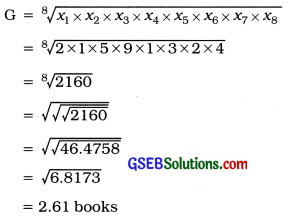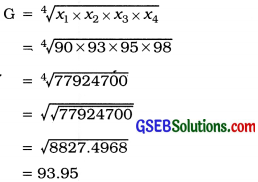# GSEB Solutions Class 11 Statistics Chapter 3 Measures of Central Tendency Ex 3.3

Gujarat Board Statistics Class 11 GSEB Solutions Chapter 3 Measures of Central Tendency Ex 3.3 Textbook Exercise Questions and Answers.

## Gujarat Board Textbook Solutions Class 11 Statistics Chapter 3 Measures of Central Tendency Ex 3.3

Question 1.
The following data show the number of books read by 8 students of a class during last month:
2, 1, 5, 9, 1, 3, 2, 4
Find the average number of books read using geometric mean.
Here, x1 = 2; x2 = 1: x3 = 5; x4 = 9; x5 = 1; x6 = 3; x7 = 2 and x8 = 8Hence, mean of books read = 2.61 books.
[Note: To find 8th root of a number, find ( the square root again of square root ; found, first, repeat this process till the last square roots. Here, square root process is done thrice.]

Question 2.
The value of a machine depreciates at the rate of 10%, 7%, 5% and 2% In Its first four years respectively. Find the average rate of depreciation using an appropriate method.
Here, the depreciation values of a machine are given in percentage. Therefore, an appropriate average of depreciation Is geometric mean.

Considering the percentage depreciation of value of a machine, we get the following observation :
x1 = 100 – 10 = 90;
x2 = 100 -7 = 93;
x3 = 100 – 5 = 95;
x4 = 100 – 2 = 98

Average of depreciation:Hence, average rate of depreciation is four years = (100 – 93.95) = 6.05 %.Question 3.
A taxi travelled 15 km on Monday and 254 km on Tuesday. Find the average distance travelled over these two days using geometric mean.
G = $$\sqrt{x_{1} \times x_{2}}$$
= $$\sqrt{15 \times 254}$$
= $$\sqrt{3810}$$